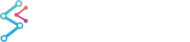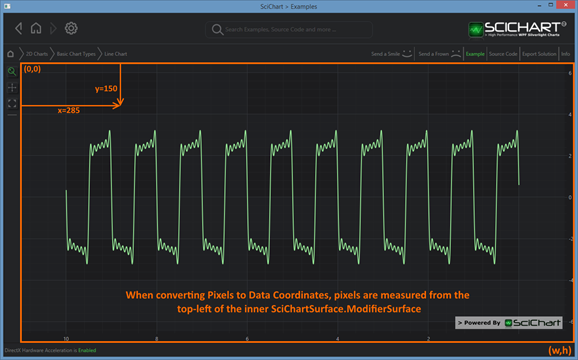﻿ Axis APIs - Convert Pixel to Data Coordinates | WPF Chart DocumentationSciChart WPF 2D Charts > Axis APIs > Axis APIs - Convert Pixel to Data Coordinates
Axis APIs - Convert Pixel to Data Coordinates

SciChart provides a clean and simple API to transform pixels to data-values and vice versa via the AxisBase.GetCurrentCoordinateCalculator() method.

## Where Pixel Coordinates are measured from

It is important to note when converting Pixels to Data Coordinates and vice versa that pixels are measured from the top-left inside corner of the chart. So, the pixel coordinate (0,0) corresponds to the data-value at (XAxis.VisibleRange.Min, YAxis.VisibleRange.Max), and the pixel coordinate (width, height) corresponds to the data-value at (XAxis.VisibleRange.Max, YAxis.VisibleRange.Min).Where Pixel Coordinates are Measured From with the CoordinateCalculator API

## Getting a CoordinateCalculator instance

AxisBase.GetCurrentCoordinateCalculator() returns a coordinate calculator instance which is valid for the current render pass.

Note: If the Axis.VisibleRange changes, the data changes, or the viewport size changes, then the CoordinateCalculator will give incorrect results. It is advisable to cache the Coordinate Calculator only for a short period of time, e.g. when using inside a loop.

## Converting from Data to Pixels

Data-values are converted to pixel coordinates via the ICoordinateCalculator.GetCoordinate() method.

#### Converting NumericAxis Data to Pixels

Converting NumericAxis Data to Pixels
Copy Code
```// Get coordinate from a NumericAxis
NumericAxis numericAxis = new NumericAxis();
var coordCalc = numericAxis.GetCurrentCoordinateCalculator();
// GetCoordinate expects a double value. You can cast to double if your data is integer
double pixelCoord = coordCalc.GetCoordinate(1.23d);
```

#### Converting TimeSpanAxis Data to Pixels

Converting TimeSpanAxis Data to Pixels
Copy Code
```// Get coordinate from a TimeSpanAxis
NumericAxis numericAxis = new NumericAxis();
var coordCalc = numericAxis.GetCurrentCoordinateCalculator();
// GetCoordinate expects a double value. You can pass in TimeSpan.Ticks
double pixelCoord = coordCalc.GetCoordinate(TimeSpan.FromMilliseconds(1234).Ticks);
```

#### Converting DateTimeAxis Data to Pixels

Converting DateTimeAxis Data to Pixels
Copy Code
```// Get coordinate from DateTimeAxis
DateTimeAxis dateTimeAxis = new DateTimeAxis();
var coordCalc = numericAxis.GetCurrentCoordinateCalculator();
// GetCoordinate expects a double value. You can pass in DateTime.Ticks
double pixelCoord = coordCalc.GetCoordinate(new DateTime(2015, 01, 01).Ticks);
```

#### Converting CategoryDateTimeAxis Data to Pixels

CategoryDateTimeAxis is a little different. GetCoordinate expects an integer, which is the index to the dataSeries.XValues. You can convert between DateTime and index and then to pixel as follows:

Converting CategoryDateTimeAxis Data to Pixels
Copy Code
```// Get coordinate from CategoryDateTimeAxis
CategoryDateTimeAxis catAxis = new CategoryDateTimeAxis();
ICategoryCoordinateCalculator coordCalc = catAxis.GetCurrentCoordinateCalculator() as
ICategoryCoordinateCalculator;
int index = coordCalc.TransformDataToIndex(new DateTime(2015, 01, 01));
double pixelCoord = coordCalc.GetCoordinate(index);
```

#### Converting NumericAxis Pixels to Data

Converting NumericAxis Pixels to Data
Copy Code
```// Get data-value from a coordinate with NumericAxis
NumericAxis numericAxis = new NumericAxis();
var coordCalc = numericAxis.GetCurrentCoordinateCalculator();
// GetDataValue expects a pixel coordinate and returns double
// You can cast the return value if your data is integer
Point mousePoint;
double dataValue = coordCalc.GetDataValue(mousePoint.X);
```

#### Converting TimeSpanAxis Pixels to Data

Converting TimeSpanAxis Pixels to Data
Copy Code
```// Get data-value from a coordinate with TimeSpanAxis
TimeSpanAxis timeSpanAxis = new TimeSpanAxis();
var coordCalc = timeSpanAxis.GetCurrentCoordinateCalculator();
// GetDataValue expects a pixel coordinate and returns double
// You need to wrap the double in a TimeSpan to complete the conversion
Point mousePoint;
double dataValueAsDouble = coordCalc. GetDataValue (mousePoint.X);
TimeSpan dataValue = new TimeSpan((long) dataValueAsDouble);
```

#### Converting DateTimeAxis Pixels to Data

Converting DateTimeAxis Pixels to Data
Copy Code
```// Get data-value from a coordinate with DateTimeAxis
DateTimeAxis dateTimeAxis = new DateTimeAxis ();
var coordCalc = dateTimeAxis.GetCurrentCoordinateCalculator();
// GetDataValue expects a pixel coordinate and returns double
// You need to wrap the double in a DateTime to complete the conversion
Point mousePoint;
double dataValueAsDouble = coordCalc.GetDataValue(mousePoint.X);
DateTime dataValue = new DateTime ((long)dataValueAsDouble);
```

#### Converting CategoryDateTimeAxis Pixels to Data

CategoryDateTimeAxis is a little different. GetDataValue returns an integer, which is the index to the dataSeries.XValues. You can convert between pixel to index and then to DateTime as follows:

Converting CategoryDateTimeAxis Pixels to Data
Copy Code
```// Get data-value from a coordinate with CategoryDateTimeAxis
CategoryDateTimeAxis catAxis = new CategoryDateTimeAxis();
var coordCalc = catAxis.GetCurrentCoordinateCalculator();
// GetDataValue expects a pixel coordinate and returns double (which in this case is an
// index to data)
Point mousePoint;
int dataIndex = (int)coordCalc.GetDataValue(mousePoint.X);
DateTime dataValue = coordCalc.TransformIndexToData(dataIndex);
```

## Transforming Pixels to the Inner ViewportGiven a SciChartSurface bounds and a mouse coordinate (x,y), you may need to transform to viewport coordinates (x’,y’)

Given a SciChartSurface with Axis on the left, a mouse-coordinate relative to the SciChartSurface must be transformed relative to the viewport (the central area) before the CoordinateCalculator API can be used. To do this, use the following code.

Transforming Pixels to the Inner Viewport
Copy Code
```var sciChartSurface = new SciChartSurface();
sciChartSurface.PreviewMouseDown += (s,e) =>
{
// The mouse-point relative to the SciChartSurface
Point xy = e.GetPosition(sciChartSurface);

// Translates the mouse point (from root grid coords) to ModifierSurface coords
Point x1y1 = sciChartSurface.RootGrid.TranslatePoint(xy, sciChartSurface.ModifierSurface);

// you can now use this coordinate to convert to data values
double dataValue = sciChartSurface.XAxis.GetCurrentCoordinateCalculator()
.GetDataValue(x1y1.X);
};
```